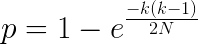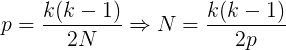# kleemans.ch

## Calculating hash lengths

February 18, 2019

TLDR; How big should a sample space be if the amount of tries & probability are fixed? (Or: How many hash-digits should I check to be somewhat sure to not have a collision?)

Expected tries:
Max. collision probability (in %):
Min. space of possible values:
Example of random hash:
(better collision probability approx.: {{hashLengthCtrl.prob}})

Last week at work, I worked on some piece of code and everytime after a change, a new build artifact would be assembled which I had to test.

They would look like this:

• build_760aa1cd.zip
• build_ef08028e.zip
• build_081b0505.zip

with the last part being some kind of random hash (of the SHA family). Now for testing I had to be sure that I wouldn't pick an old build and I just memorized the first three letters of the random hash, for example "build_ef0".

I then wondered, how many digits should I have to memorize for being reasonably certain that I wouldn't have a "collision" with an already existing build artifact? I was searching for how big the sample space should be.

Jeff Preshing wrote a nice post about Hash Collision Probabilities, and it contains the following formula:where

• p: probability that at least two of them are equal
• k: number of randomly generated values
• N: the sample space

With the simplified versions, we can easily calculate how big the space should be:Solving for N, we now know:

If you expect to compare 50 different hashes and you want to have a maximum collision probability of 20%, you should have a space of at least 6125, or 3.15 hexadecimal hash digits.

Which means that my initial 3 digits are just not enough to get a collision in only 20% of the time. In reality, if one would pick 50 random 3-digit hashes, the collision probability would be around 26%.

You can punch in some other numbers in the calculator at the beginning of this post. The sample space is an approximation (which performs good for low probabilities, see Jeff's post), the better probability approximation of having a collision is calculated separately below the calculator.

Happy hacking!